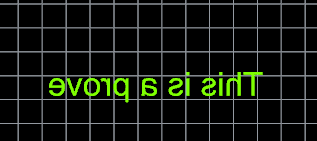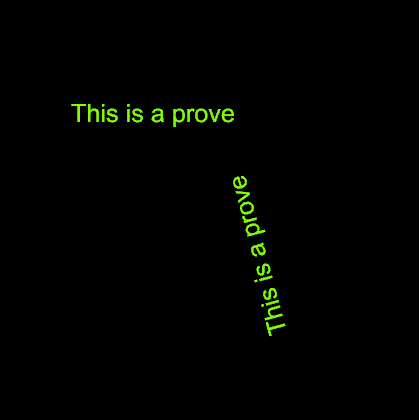# Inverted Text - mirror - c#

I have an inverted text with the mirror command, I want to put it normal again but without moving it from the position where it is-

Ex.I want to put it “This is a prove” without move his center

Code C#

Are you using Rhino 5 or 6? RhinoCommon or C++?

– Dale

1 Like

Hi Dale,

Rhino 5, c#

``````protected override Result RunCommand(RhinoDoc doc, RunMode mode)
{
var go = new GetObject();
go.SetCommandPrompt("Select text to flip");
go.SetCustomGeometryFilter(TextObjectGeometryFilter);
go.Get();
if (go.CommandResult() != Result.Success)
return go.CommandResult();

var obj_ref = go.Object(0);
var text_obj = obj_ref.Object() as TextObject;
if (null == text_obj)
return Result.Failure;

var bbox = text_obj.Geometry.GetBoundingBox(true);
var p0 = (bbox.Corner(false, true, true) + bbox.Corner(true, true, true)) / 2.0;
var p1 = (bbox.Corner(false, false, true) + bbox.Corner(true, false, true)) / 2.0;
var plane = text_obj.TextGeometry.Plane;
var dir = Vector3d.CrossProduct(p1 - p0, plane.Normal);
var xform = Transform.Mirror(p0, dir);
plane.Transform(xform);

var text = text_obj.TextGeometry.Duplicate() as TextEntity;
text.Plane = plane;

doc.Objects.Replace(obj_ref, text);
doc.Views.Redraw();

return Result.Success;
}

private bool TextObjectGeometryFilter(RhinoObject rhObject, GeometryBase geometry, ComponentIndex ci)
{
return null != rhObject && null != rhObject as TextObject;
}
``````

– Dale

Hi Dale, this idea is almost perfect, I explain you

This is the text after to do a mirror command,The text was put in normal sense, but tilt angle cannot be changed and it should remain so…,I’m running into the same issue currently. Did you manage to solve this?

@dale Is there a solution for this?

Hi Dale,

I’m afraid this won’t work. I think I need to have control over that option individually.

I have objects like these: where I use the center of the line to position the center of the text and rotate it along the angle of the line such that the bottom of the text is on the line.

If I turn that option on it gets automatically rotated in the other direction, which is not what we would like.

This is what I get with normal Mirror command (yellow line is mirror line, the one in the bottom left is the original) Both texts are in correct position and rotation but the mirrored texts are backwards.

This is how it looks like when I turn on that option such that text reads forward when viewed from behind:

The mirrored copy on the right is correct, the original object and the mirror copy on the top are wrong

And this is what I’m trying to do by creating a new mirror command

210212 MirrorError.3dm (26.0 KB)

@dale Any idea how to solve this or where I should look into?

Does setting this annotation style option provide you the result you want? This can be assigned on a per-text object basis, by the way.

– Dale

Actually, looking at my own example, it probably won’t.

I was thinking maybe this could work but I haven’t tested it yet:

• Check the plane of the TextEntity
• Find intersection point between the X or Y-axis of that plane and the line to mirror
• Use that intersection point to calculate the angle to the mirror line and rotate the TextEntity double that angle

Not sure if my blurry drawing helps:

I can already see that I’ll run into issues figuring out which axis of the plane it should take in which case but if I could figure that out I think it could work. Do you have any other suggestions?

I think you could also achieve this with remapping the geometry (plane to plane)
quick and dirty example:

``````from Rhino.Geometry import *
import Rhino
import rhinoscriptsyntax as rs
import scriptcontext as sc
from math import pi as pi

objects = rs.GetObjects("select objects to mirror")

mp = Plane(Point3d(mirrorline), Vector3d(mirrorline-mirrorline), Plane.WorldXY.ZAxis)

bb = rs.BoundingBox(objects)

objects = [rs.coercegeometry( object) for object in objects]

bb0 = bb
bb2 = bb

cen = (bb0+bb2)/2

plane = Plane(cen, Plane.WorldXY.ZAxis)

mirrorplane = Plane(cen, Plane.WorldXY.ZAxis)

transform = Transform.Mirror(mp)
mirrorplane.Transform(transform)

transform = Transform.Rotation(pi, mirrorplane.XAxis, mirrorplane.Origin)
mirrorplane.Transform(transform)

transform = Transform.PlaneToPlane(plane, mirrorplane)

for object in objects:
object.Transform(transform)
sc.doc.Views.Redraw()``````
1 Like

Thanks @Gijs ! I’ll test this when I have some time

Hi Dale,

What is the API command/property for this toggle? I have a workflow that need to put this toggle in a script.

Many thanks!
Vincent

Edit:
Never mind. I finally find it. For people who might need this, it is `DimensionStyle.DrawForward Property`.